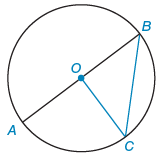Alyssa Z.

# Given:     In ⊙O, OC ⊥ AB and OC = 9. Find the following. (a) AB   (b) BC

Consider the following figure.A circle has center point O. There are 3 points on the circle and 3 interior line segments.

1. Point A is on the lower left.
2. Point B is on the upper right.
3. Point C is on the lower right.
4. Segment A B goes from A up and right to B, passing through O.
5. Segment B C goes from B down and slightly left to C.
6. Segment O C goes from O down and right to C.

Given:     In ⊙OOC ⊥ AB and OC = 9.

Find the following.

(a) AB

(b) BC